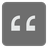Max Digit
Elementary
English PL RU

You have a number and you need to determine which digit in this number is the biggest.

Input: A positive int.

Output: An Int (0-9).

Example:

```max_digit(0) == 0
max_digit(52) == 5
max_digit(634) == 6
max_digit(1) == 1
max_digit(10000) == 1
```